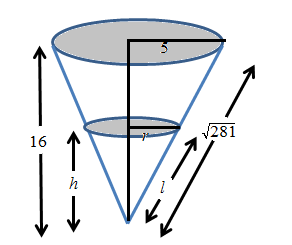# To find the rate of liquid being poured in.### Single Variable Calculus: Concepts...

4th Edition
James Stewart
Publisher: Cengage Learning
ISBN: 9781337687805### Single Variable Calculus: Concepts...

4th Edition
James Stewart
Publisher: Cengage Learning
ISBN: 9781337687805

#### Solutions

Chapter 4, Problem 23P
To determine

## To find the rate of liquid being poured in.

Expert Solution

11.204 cm3/min

### Explanation of Solution

Given:

A container in the shape of inverted cone has height 16 cm and radius 5 cm at the top.

Calculation:

The diagram is show below:From the diagram,

r5=h16r=5h16

The volume of the cone is

V=13πr2h=13π(5h16)2h=25π768h3

Differentiate the above equation with respect to t on both sides.

dVdt=25π768(3h2)dhdtdVdt=25π256(h2)dhdt ...... (1)

But the rate of change of volume is also equals to the difference of what is being added ( 2 cubic centimeter/ min) and what is oozing out ( kπrl , where πrl is the area of the cone and k is proportional constant).

So,

dVdt=2kπrl ...... (2)

From equations (1) and (2), equate right hand side expressions

25π256(h2)dhdt=2kπrl

Substitute dhdt=0.3,r=5(10)16=258

l281=1016l=58281

Then

25π256(102)(0.3)=2kπ(258)58281125kπ28164=2+750π256

Solve for variable k,

k=256+375π250π281

If height of the liquid is constant, then the rate of oozing, kπrl must be equal to rate of liquid being poured in.

That is

dVdt=kπrldVdt=256+375π250π281π(258)(52818)=11.204 cm3/min

### Have a homework question?

Subscribe to bartleby learn! Ask subject matter experts 30 homework questions each month. Plus, you’ll have access to millions of step-by-step textbook answers!